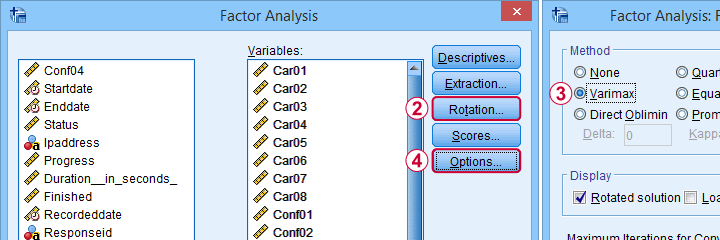SPSS TUTORIALS BASICS ANOVA REGRESSION FACTOR CORRELATION

# Overview SPSS Factor Analysis Tutorials## Factor Analysis in SPSS

### SPSS Factor Analysis – Beginners Tutorial

Factor analysis examines which variables in your data measure which underlying factors.

This tutorial illustrates the ideas behind factor analysis with a simple step-by-step example in SPSS.

### SPSS Factor Analysis – Intermediate Tutorial

A nicely detailed, step-by-step SPSS factor analysis example.

We'll cover how & why to use promax rotation, remove items, decrease the number of factors and more...

### APA Reporting SPSS Factor Analysis

This tutorial quickly walks you through some handy routines for creating APA style tables for

## Computing Scale Scores in SPSS

### Cronbach’s Alpha in SPSS

This tutorial quickly walks you through the basics as well as troubleshooting such as

• increasing Cronbach’s alpha by removing items;
• fixing a negative Cronbach’s alpha;
• using pairwise exclusion of missing values;
• APA style reporting.

### Split-Half Reliability in SPSS

Split-half reliability is the adjusted correlation between two test halves.

This tutorial quickly walks you through

• a quick data screening;
• the SPSS reliability dialogs;
• the resulting output;
• the Spearman-Brown and Horst formulas.

### How to Compute Means in SPSS?

The right way to compute means over variables is SPSS’ MEAN function.

For example: COMPUTE MEAN_SCORE = MEAN(Q1 TO Q5). computes mean_score as the mean over variables Q1 to Q5. This method also works correctly if you there's any missing values in your data.

## Factor Analysis - Background Tutorials

### Covariance – Quick Introduction

A covariance is basically an unstandardized correlation.

This tutorial covers

• when and why to use covariances instead of correlations;
• basic formulas for covariances;
• obtaining covariances from Googlesheets and SPSS;
• and way more...

### SPSS FACTOR Computes Wrong Covariances

SPSS FACTOR computes wrong covariances when pairwise exclusion of missing values is used.

For correct covariances, use

• CORRELATIONS or (better)
• REGRESSION.